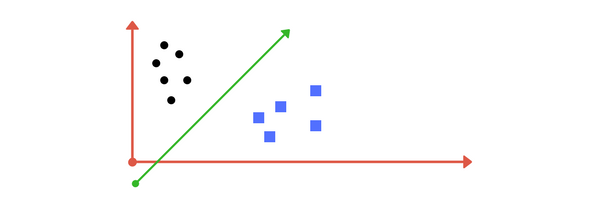What is Support Vector Machine (SVM)?

# What is Support Vector Machine (SVM)?

Support Vector Machine is a supervised learning algorithm which can be used for both classification or regression problems. In this algorithm, data points are plotted in an n-dimension space (where n is a number of features) with the value of each feature being the value of a particular coordinate. Then, classification is done by finding hyper-plane separating between two classes. Support Vector Machine is a frontier which best segregates the two classes (hyper-plane/ line).

Support Vector Machine is a machine learning technique widely used in pattern recognition, object classification and regression analysis.

Support Vector Machine is a machine learning technique widely used in pattern recognition, object classification and regression analysis. Given a set of training data belonging to different classes, SVM attempts to derive a hyper plane or a set of hyper planes that form the boundary between the data belonging to different classes. Any test data can be classified to one of the predefined classes by checking its position relative
to the hyper planes.Read these articles from analyticsvidhya and medium for detail understanding.